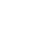Introduction

A pendulum is a mass on the end of a string of negligible mass, which, when initially displaced, will swing back and forth under the influence of gravity over its lowest point. The regular motion of a pendulum can be used for timekeeping.

Problem

To identify the relationships between the frequency of a simple pendulum and its mass, amplitude and length.

Variables:

Independent: Amplitude, mass, string length
Dependent: Frequency
Controlled: Timer, testing apparatus, type of string, clamp, etc.

Materials

·    Utility Stand
·    Clamp
·    Test- Tube Clamp
·    Split Rubber Stop
·    String
·    Stopwatch
·    Metre stick
·    Metal masses (50g, 100g, and 200g)

Method

1)    A data table was created to record information.
2)    Materials were collected and brought to the workstation.
3)     A string of 110cm was obtained and a 200g mass was attached to one end. It was placed into the split rubber stopper and adjusted to 100cm.  It was then clamped so it would not slip.
4)    The pendulum was set at an amplitude of 10cm and released. The timer recorded the amount of time taken for it to complete 20 cycles. Tests were repeated 2-3 times to ensure accuracy and frequency.
5)    Step 4-5 were repeated using amplitudes of 20cm and 30cm. Data was recorded.
6)    Then the timer recorded the amount of time required for 20 complete cycles of the pendulum using a pendulum length of 100cm, an amplitude of 10cm, and 50g, 100g, and 200g masses.  Data was recorded.
7)    Finally the timer recorded the amount of time required to complete 20 complete cycles of the pendulum using an amplitude of 10cm and a constant mass. Length of 100cm, 80cm, 60cm, 40cm, and 20 cm were timed and recorded.

Diagram 1a: Pendulum apparatus similar in function to the one used in the experiment.

Observation Table

Δ Amplitude

Δ Mass

Δ String Length

Discussion

In both the Frequency vs. Amplitude (100g) and Mass vs. Frequency (Amp: 20cm) the slope of the line remained constant. This means there is no correlation between the frequency and the amplitude or mass attached to the pendulum. It will not matter if we adjust either of those two variables in any shape or form, the frequency will remain constant.

However, with the Length vs. Frequency, there is a negative correlation between the frequency and length. This means by increasing the length of the pendulum decreases the frequency and vice versa. This is the only factor affecting the frequency of the pendulum and is apparent through qualitative and quantities observations.

The frequency and length of the pendulum string are inversely related to each other via the square root of the length.

Subscribe
Notify of